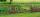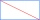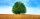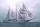# Right triangle + length - math problems

#### Number of problems found: 347

• Cylinder horizontallyThe cylinder with a diameter of 3 m and a height/length of 15 m is laid horizontally. Water is poured into it, reaching a height of 60 cm below the axis of the cylinder. How many hectoliters of water is in the cylinder?
• DiagonalsDraw a square ABCD whose diagonals have a length of 6 cm
• Spruce heightHow tall was spruce that was cut at an altitude of 8m above the ground and the top landed at a distance of 15m from the heel of the tree?
• Body diagonalCuboid with base 7cm x 3,9cm and body diagonal 9cm long. Find the height of the cuboid and the length of the diagonal of the base,
• DecagonCalculate the area and circumference of the regular decagon when its radius of a circle circumscribing is R = 1mThe ladder has a length 3.5 meters. He is leaning against the wall so that his bottom end is 2 meters away from the wall. Determine the height of the ladder.
• BuildingHow high is the building that throws horizontal shadow 95.4 m long at angle 50°?
• Three pointsThree points A (-3;-5) B (9;-10) and C (2;k) . AB=AC What is value of k?
• AcreageWhat acreage has a rectangular plot whose diagonal is 34 meters long and one side has a length of 16 meters. ?
• LighthouseMarcel (point J) lies in the grass and sees the top of the tent (point T) and behind it the top of the lighthouse (P). | TT '| = 1.2m, | PP '| = 36m, | JT '| = 5m. Marcel lies 15 meters away from the sea (M). Calculate the lighthouse distance from the sea
• Diagonal to areaCalculate the area of a rectangle in which the length of the diagonal is 10 cm.
• Hexagon cut pyramidCalculate the volume of a regular 6-sided cut pyramid if the bottom edge is 30 cm, the top edge us 12 cm, and the side edge length is 41 cm.
• Square diagonalCalculate the length of the square diagonal if the perimeter is 476 cm.
• Free space in the gardenThe grandfather's free space in the garden was in the shape of a rectangular triangle with 5 meters and 12 meters in length. He decided to divide it into two parts and the height of the hypotenuse. For the smaller part creates a rock garden, for the large
• Perimeter and diagonalThe perimeter of the rectangle is 82 m, the length of its diagonal is 29 m. Find the dimensions of the rectangle.
• The stormThe top of the 5 m high mast deviated by 1 m from the original vertical axis after the storm. What is the peak now? Round to 2 decimal places.
• Floating barrelBarrel (cylinder shape) floats on water, top of the barrel is 8 dm above water, and the width of surfaced barrel part is 23 dm. Barrel length is 24 dm. Calculate the volume of the barrel.
• Two chordsCalculate the length of chord AB and perpendicular chord BC to circle if AB is 4 cm from the center of the circle and BC 8 cm from the center of the circle.
• Parametric formCalculate the distance of point A [2,1] from the line p: X = -1 + 3 t Y = 5-4 t Line p has a parametric form of the line equation. ..
• SeaHow far can you see from the ship's mast, whose peak is at 14 meters above sea level? (Earth's radius is 6370 km).

Do you have an interesting mathematical word problem that you can't solve it? Submit a math problem, and we can try to solve it.

We will send a solution to your e-mail address. Solved examples are also published here. Please enter the e-mail correctly and check whether you don't have a full mailbox.

Please do not submit problems from current active competitions such as Mathematical Olympiad, correspondence seminars etc...

Do you want to convert length units? See also our right triangle calculator.